You need to enable JavaScript to run this app.

# 智能归因

1. 概述

2. 快速入门

• X轴为日期类型的字段目前支持的聚合粒度为日、周、月

• 图中至少有一个指标满足表达式要求，详见后文归因分析支持的表达式

• 当前分析点 / 对比时间点不为空/null/0

• 归因分析所用数据集必须是抽取数据集，暂不支持直连数据集

• 天级：至少需要有14天的连续数据

• 周级：至少需要有8周的连续数据

• 月级：至少需要有24个月的连续数据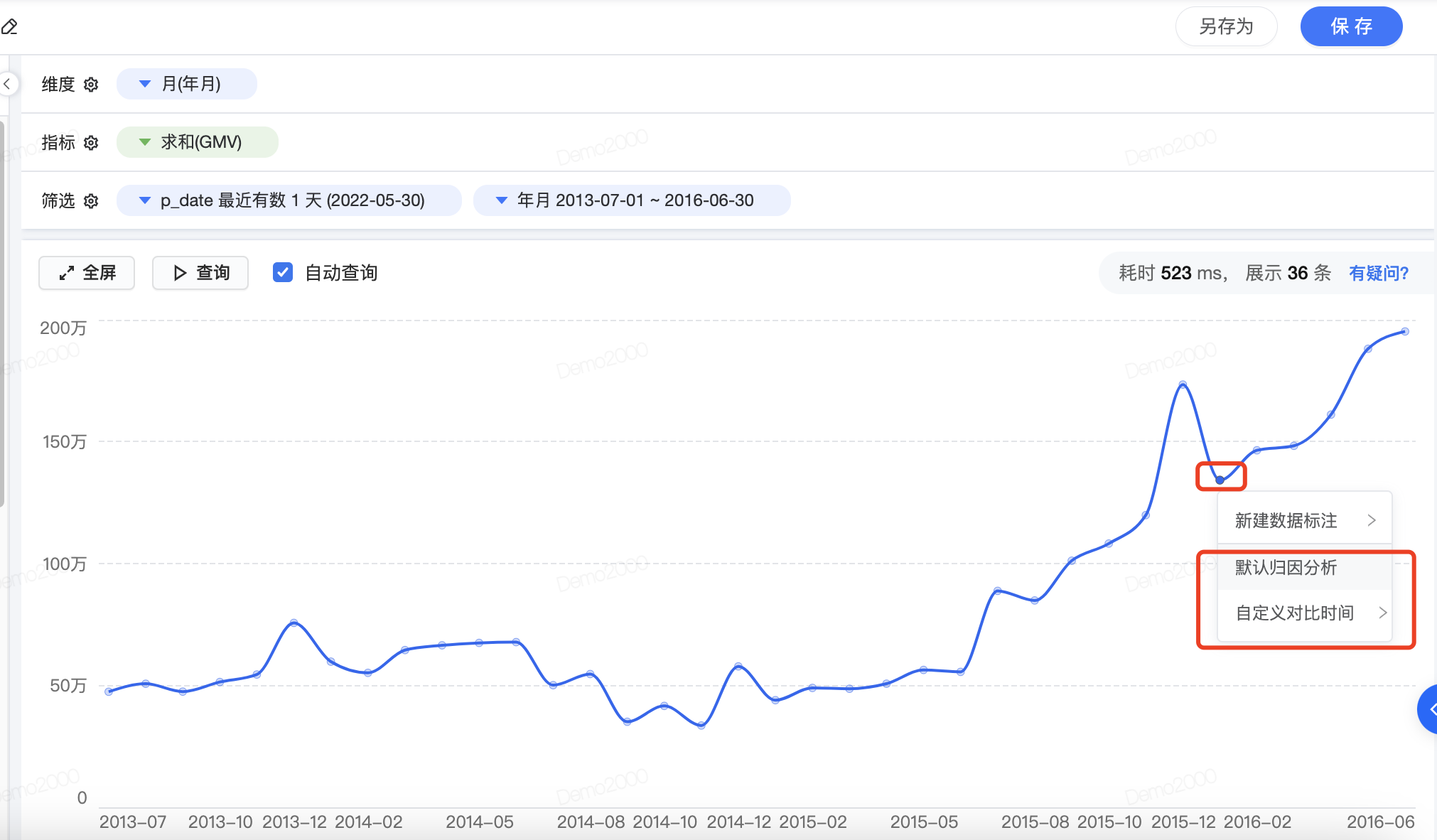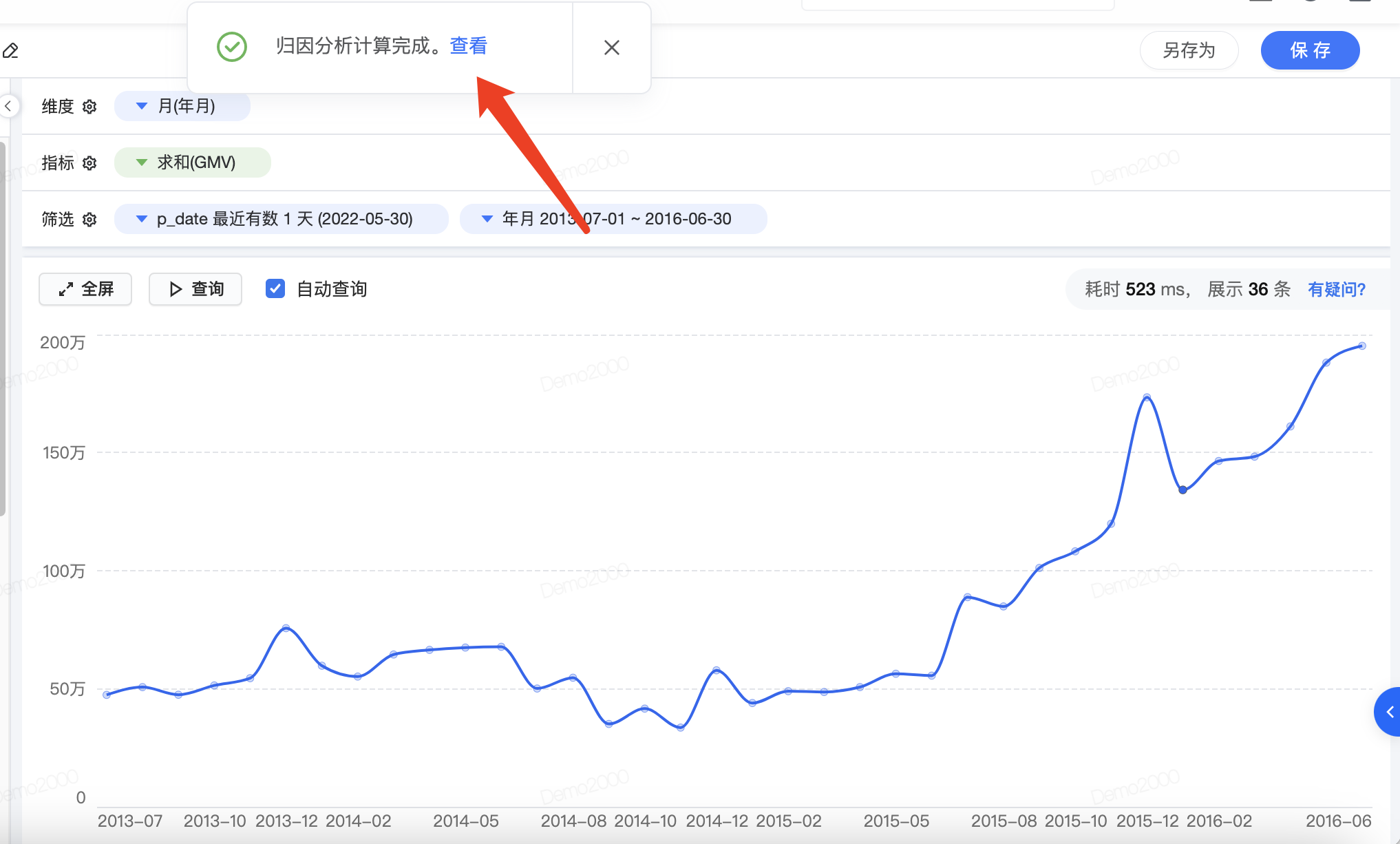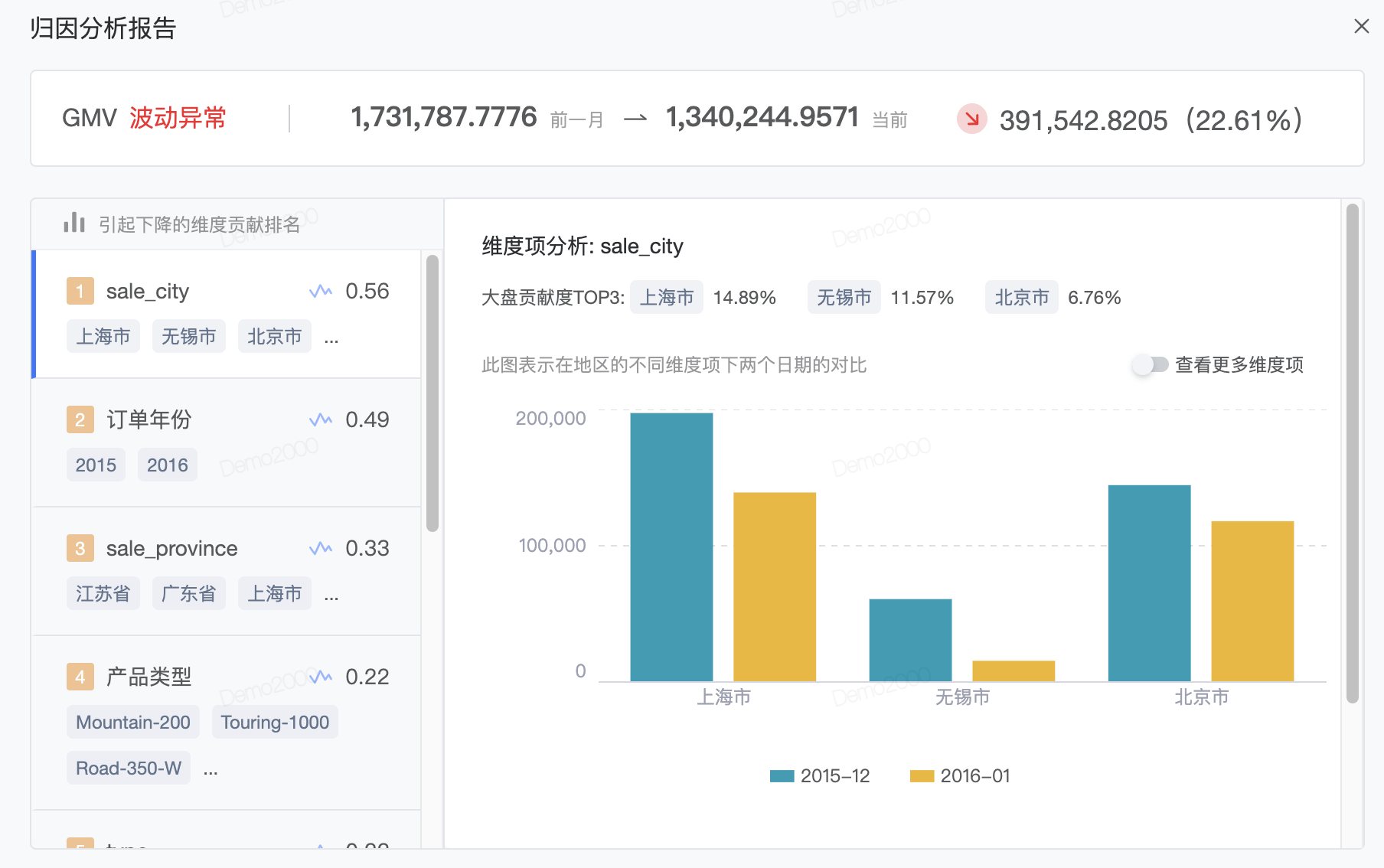3. 使用场景

## 3.1 平均延时突增问题归因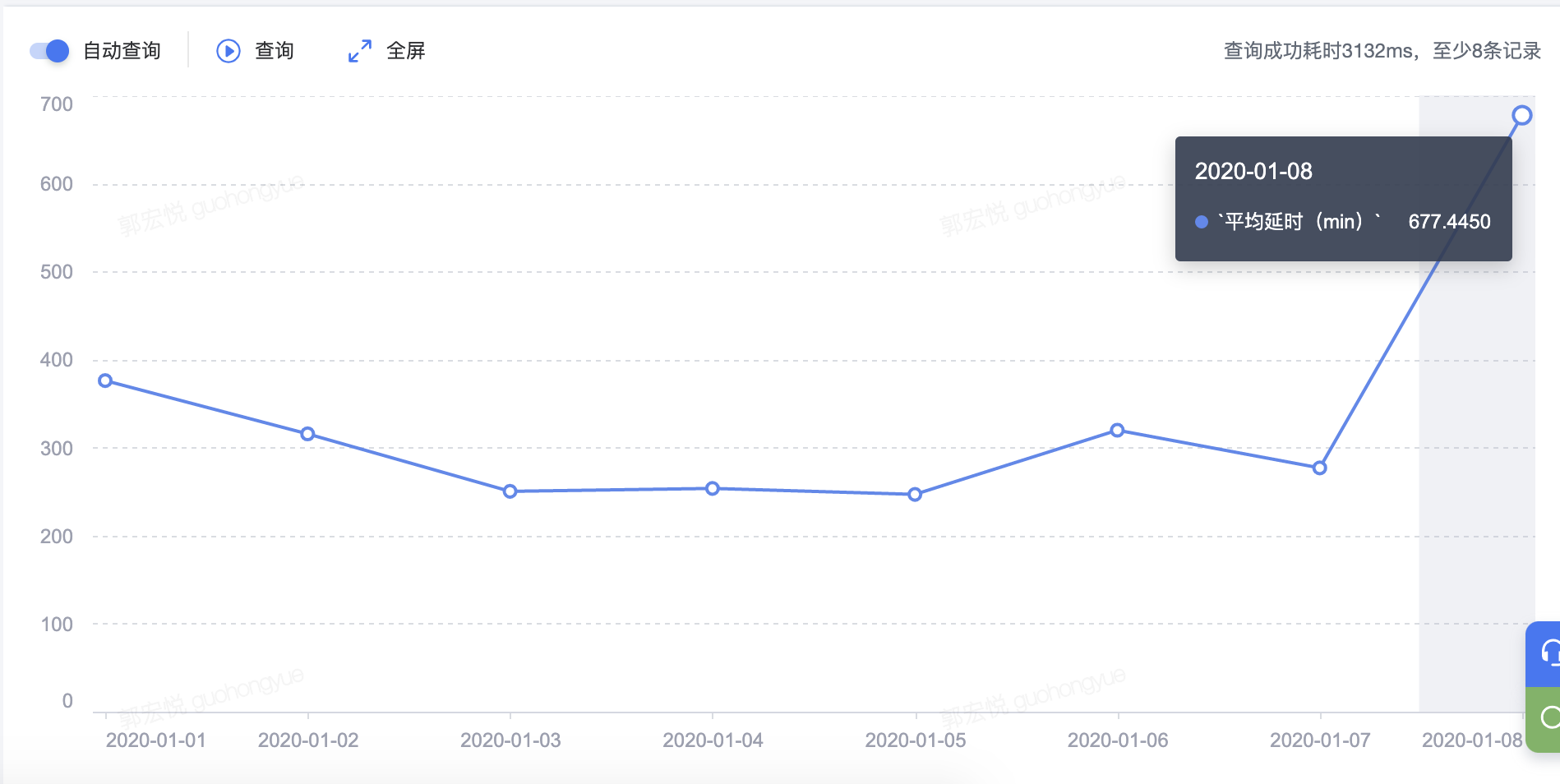## 3.2 付费金额突增问题归因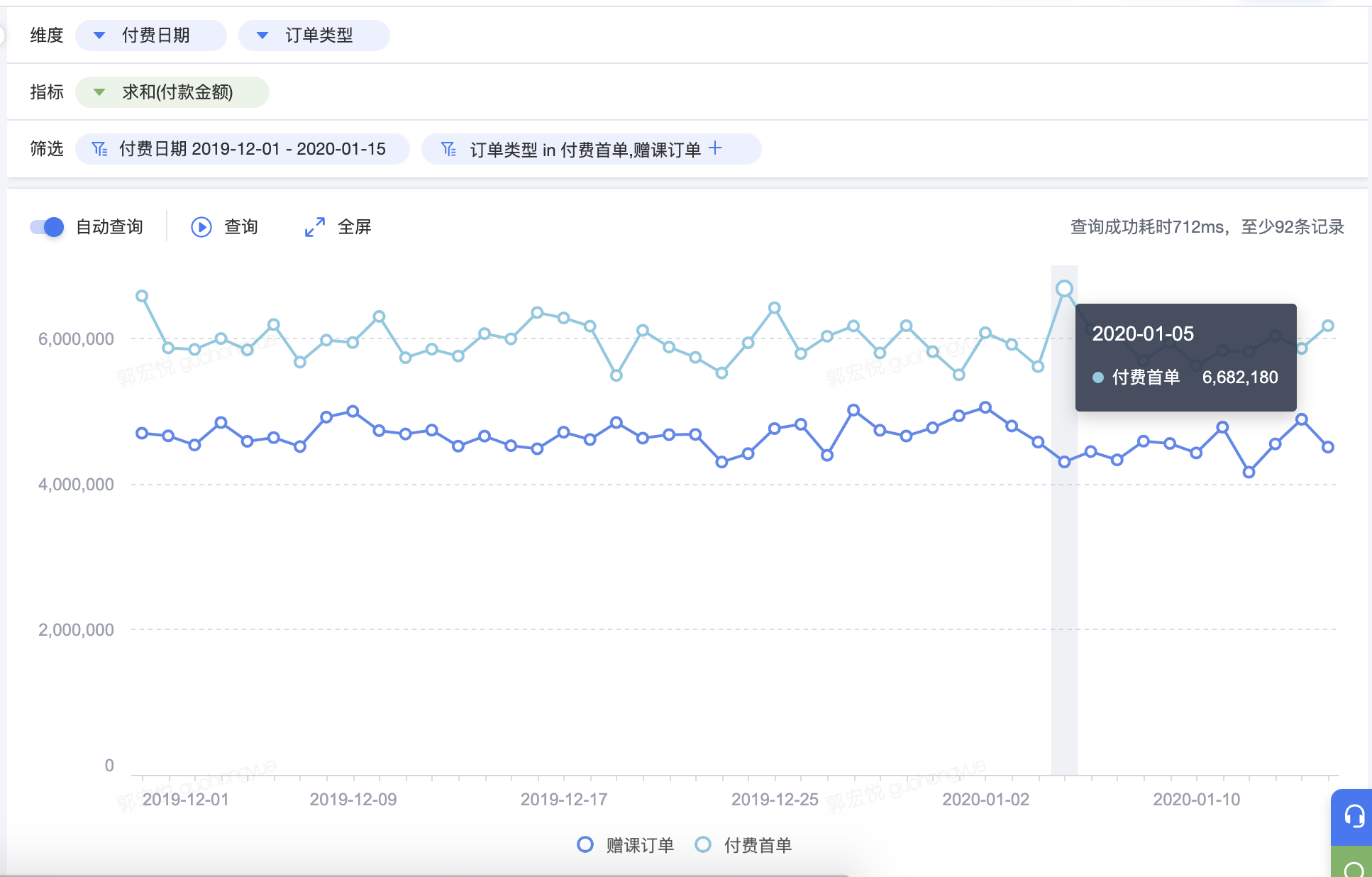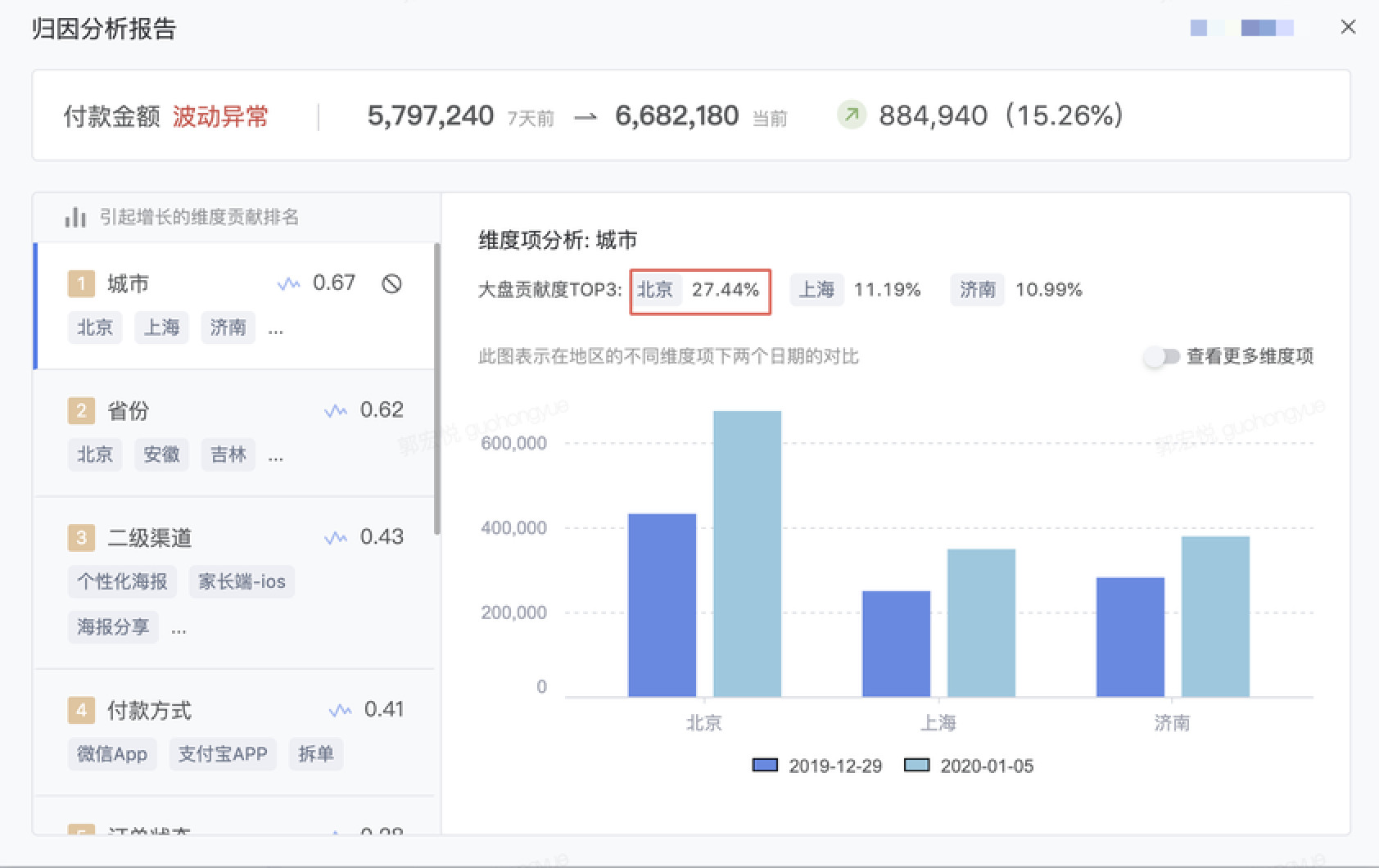4. 功能介绍

## 4.1 归因分析对比时间选择

• 默认归因分析：默认选择前一个时间节点与当前时间节点对比

• 自定义对比时间：可以自定义选择对比时间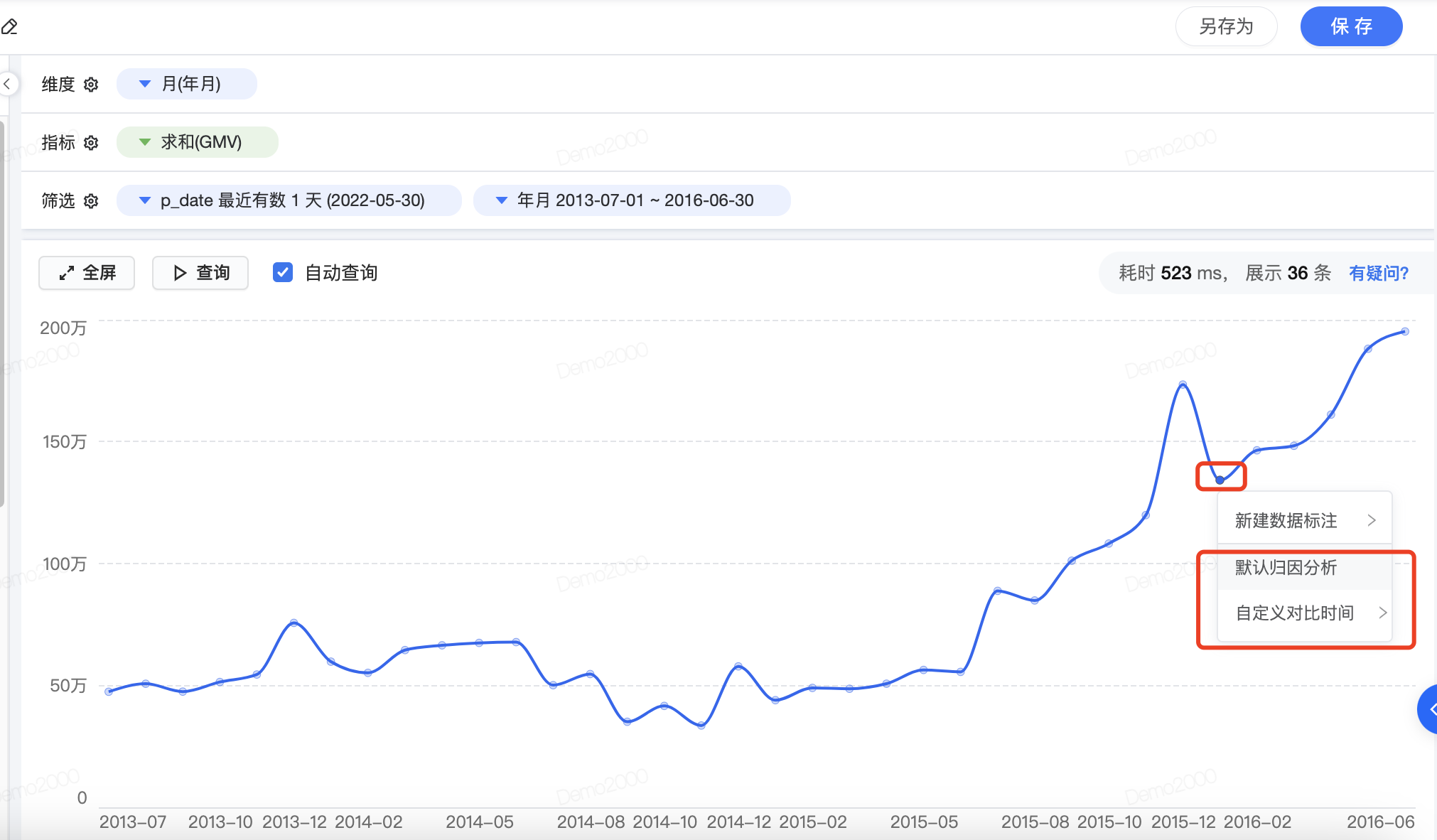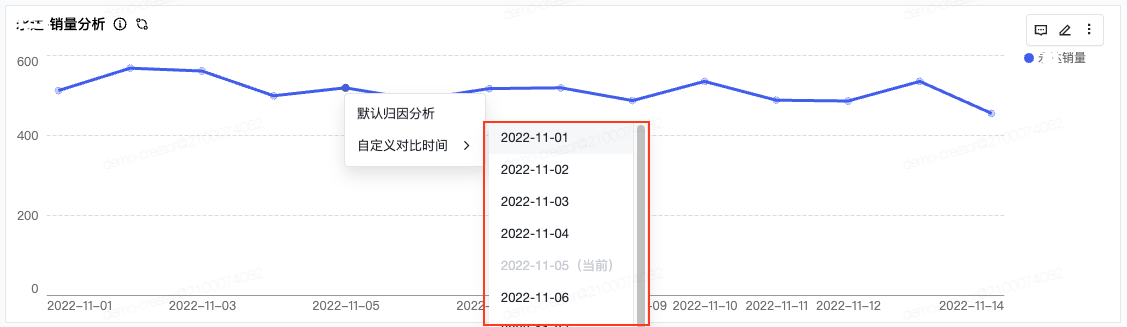## 4.2 解读归因分析报告A：波动状态，结合最近一段时间的数据，判断该指标波动是否正常
B：指标变化，相比一定时间前，指标的变化的情况
C：维度贡献排名，单个维度：

• 维度名称

• 基尼系数，用来表示对指标变化的影响程度

• 大盘贡献度TOP3维度项

• 在与CDP一起使用的情况下，此处还有新建群体的能力，详见归因建群体

D：维度的具体维度项分析

## 4.3 归因分析配置

### 4.3.1 入口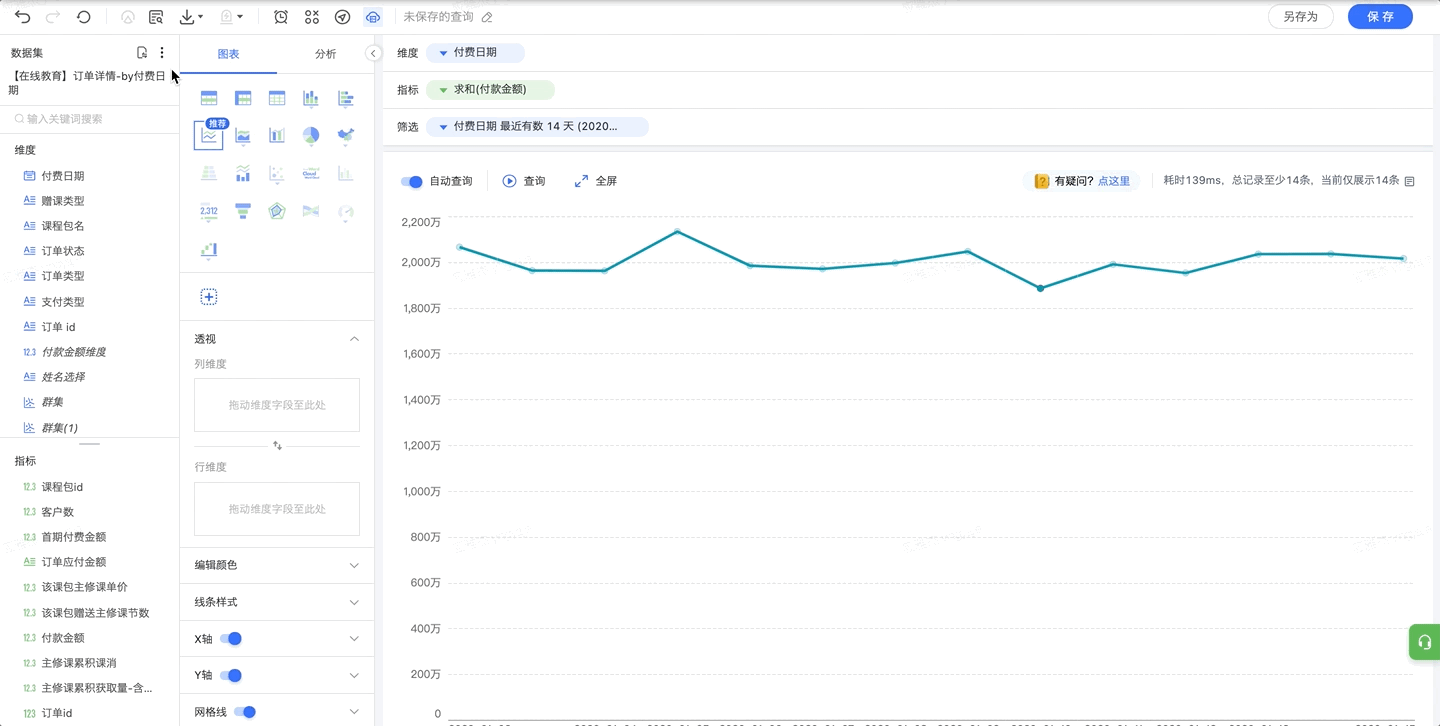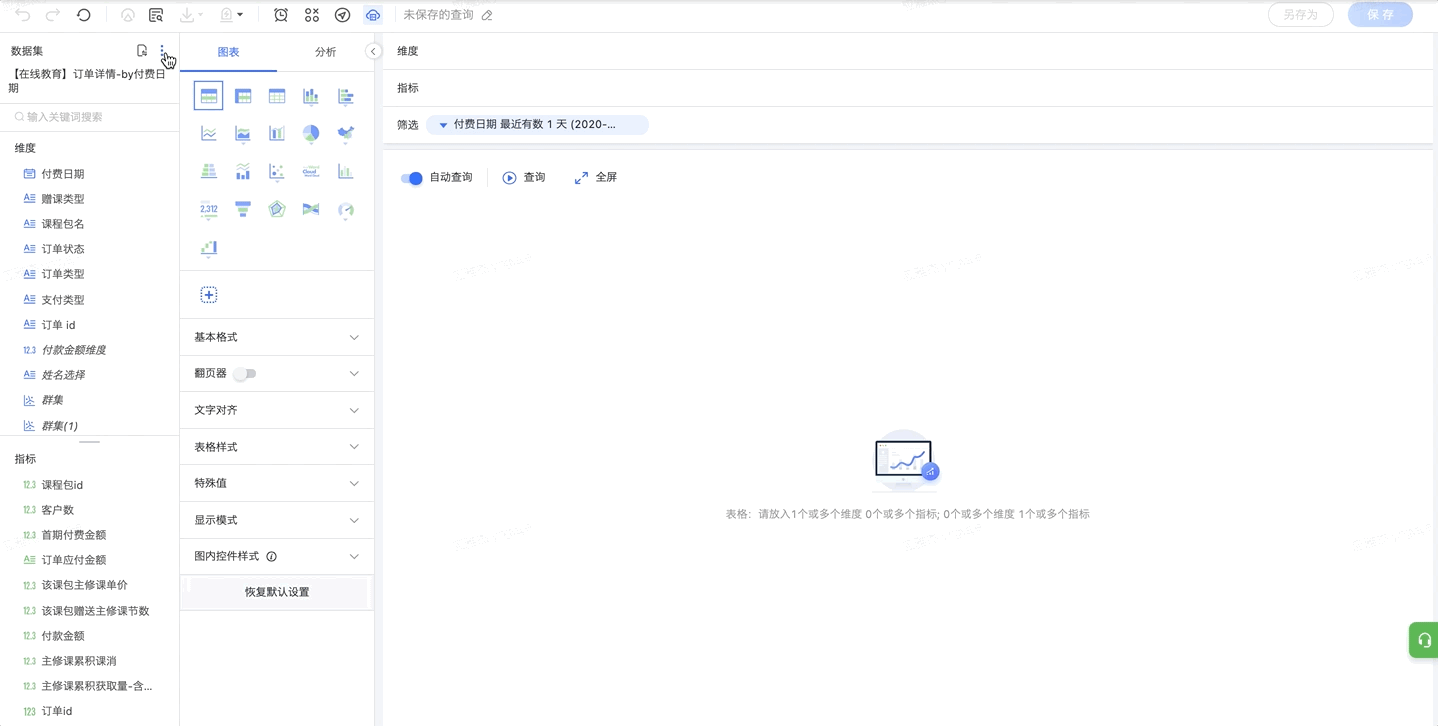### 4.3.3 异常判断依据

• 时序预测：基于系统预测自动判断，判断依据为是否符合默认时序预测的预测结果范围

• 波动大于：根据同环比波动判断，当波动幅度超过指定值时判断为异常

### 4.3.4 归因维度限制

• 维度项少于1000个才参与归因：个数可以自由设定。数据集中存在一些id、设备号等具有大量维度项，且无归因意义的维度时，可以勾选此项。

• 排除部分维度：手动排除部分维度，使之不参与归因。

## 4.4 支持的表达式

（1）归因分析支持的表达式

count(distinct XXX)count(distinct user_id)
sum(XXX)sum(col), sum(col/100), sum(1/col), sum(case when result = 'failed' then 1/result_count else 0 end)
avg(XXX)avg(col)
sum(XXX)/sum(XXX)sum(col1) / sum(col2), sum(case when result='failed' then 1 / result_count else 0 end) / sum(1 / result_count)
sum(XXX)/count(XXX)sum(vv)/count(uid)
count(XXX)count(col1)
sum(XXX)/100sum(col)/100,
100/sum(XXX)100/sum(col)
XXX/XXXcol/1, col1/col2
XXXcol

（2）归因分析不支持的表达式

count(distinct XXX) / count(distinct XXX)count(distinct pid) / count(distinct uid)
uniq(XXX) / uniq(XXX)
sum(XXX) / count(distinct XXX)sum(vv) / count(distinct uid)
sum(XXX) / sum(XXX) / sum(XXX)
sum(XXX) / sum(XXX) / 1
XXX - YYYprofit - cost

（3）后续将支持

uniq(XXX)uniq(XXX)
uniq(XXX)/100uniq(XXX)/100

## 4.5 归因的维度范围

• 某个维度表达式为id或者date；

• 某个维度的Top1维度项贡献值太小，不会显示：比如有个维度“省份”，然后上海这个维度项的贡献值最大，但是贡献百分比却 < 1%，会不显示；

• 某个维度只有一个维度项不会显示；

• 分区字段维度不会显示；

• 维度下没有维度项也不会显示；

• 在时间段分析中，若是一个维度的维度项个数过大(>10K)，不会显示；

• 当维度的数量过多的话（>15或者更多），只会取用户选取的部分维度进行加入计算（选取基尼系数高的 ，并且是当前用户最常用的维度）。